• +91 9971497814
• info@interviewmaterial.com

# Chapter 5 Complex Numbers and Quadratic Equations Ex-5.2 Interview Questions Answers

### Related Subjects

Question 1 : Find the modulus and the argument of the complex number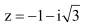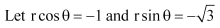On squaring and adding, we obtain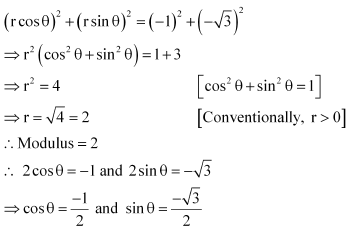Since both the values of sin θ and cos θ are negative and sinθ and cosθ are negative in III quadrant,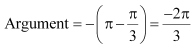Thus, the modulus and argument of the complex number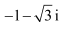are 2 and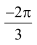respectively.

Question 2 : Find the modulus and the argument of the complex number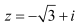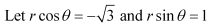On squaring and adding, we obtain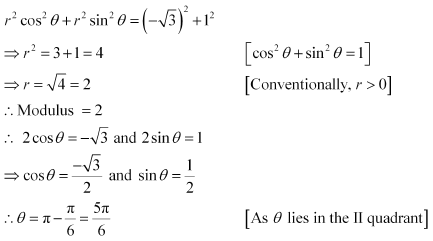Thus, the modulus and argument of the complex number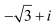are 2 and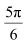respectively.

Question 3 : Convert the given complex number in polar form: 1 – i

1 – i
Let r cos θ = 1 and r sin θ = –1
On squaring and adding, we obtain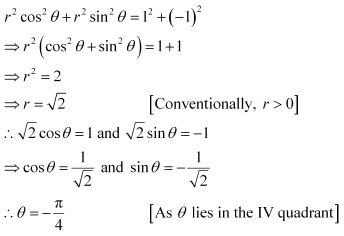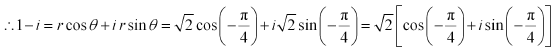This is the required polar form.

Question 4 : Convert the given complex number in polar form: – 1 + i

– 1 + i
Let r cos θ = –1 and r sin θ = 1
On squaring and adding, we obtain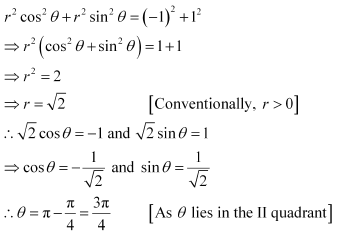It can be written,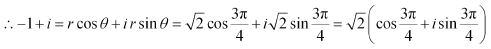This is the required polar form.

Question 5 : Convert the given complex number in polar form: – 1 – i

– 1 – i
Let r cos θ = –1 and r sin θ = –1
On squaring and adding, we obtain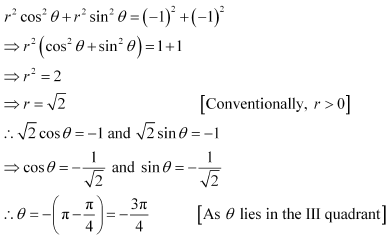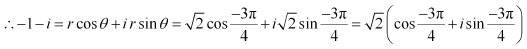This is the required polar form.

Question 6 : Convert the given complex number in polar form: –3

–3
Let r cos θ = –3 and r sin θ = 0
On squaring and adding, we obtain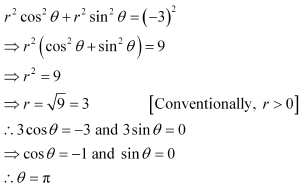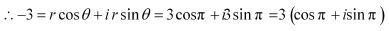This is the required polar form.

Question 7 : Convert the given complex number in polar form: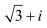Answer 7 :Let r cos θ =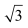and r sin θ = 1
On squaring and adding, we obtain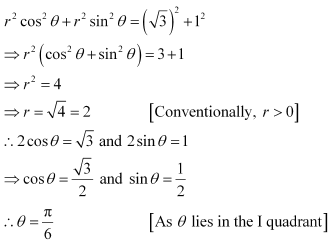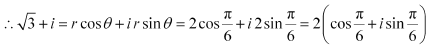This is the required polar form.

Question 8 : Convert the given complex number in polar form: i

i
Let r cosθ = 0 and r sin θ = 1
On squaring and adding, we obtain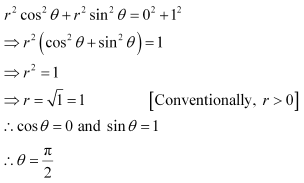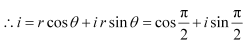This is the required polar form.

Todays Deals### Chapter 5 Complex Numbers and Quadratic Equations Ex-5.2 Contributorskrishan

Name:
Email:

# Latest News# 9000 interview questions in different categories